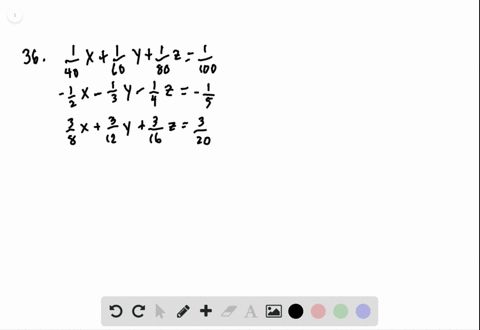Sign up for our free STEM online summer camps starting June 1st!View Summer Courses### For the following exercises, solve each system by…

02:49Other Schools

Need more help? Fill out this quick form to get professional live tutoring.

Get live tutoring
Problem 35

For the following exercises, solve each system by Gaussian elimination.
$$\begin{array}{l}{-\frac{1}{4} x-\frac{5}{4} y+\frac{5}{2} z=-5} \\ {-\frac{1}{2} x-\frac{5}{3} y+\frac{5}{4} z=\frac{55}{12}} \\ {-\frac{1}{3} x-\frac{1}{3} y+\frac{1}{3} z=\frac{5}{3}}\end{array}$$

$(5,12,15)$

## Discussion

You must be signed in to discuss.

## Video Transcript

find working good diffraction. We can win that. Pay the first equation by far so we can get a minus. Thanks. Minus fi. Why I'm blessed. 10 said good. Yo, man is 22nd question of commitment. Everything. Big drum. So he called you minus six. Thanks. And minus trend being Why on becomes ah, team sent Yu 55. And that's when we will be anything but three minus Hank Manus. Why? Gus said you got your friend so emotional. One, 23 Some would say that. Ah, no, this is, um no, we will eliminate the X now, So when we live in it, we'll have, uh, townsman to three. So I would tempt the 1st 1 We tend. Er, 1st 1 on my mind has the 3rd 1 So one kind of tree implies that we can get it. Thanks. So gonna minus for why? And blessed Nice that come true. Minus 10 to 5. Now, for the 2nd 1 we can have first when we tend with this 600 minus a 2nd 1 So six times the 1st 1 minus Nesic in one so we can get rid of the excess. Well, so man is that they minus 20 Manus Manus 20 someone is 10. Here. Why? On dhe 60 a minus fit in me. 45 exact Uncle Joe. Ah, on a gente minus for defi. Our gentle fine 1 75 So from here we see that everyone abandoned first by five. And then we do it a subjection. Does he get Ah Mann ist? And they pressed him with my honest 10. Why? And why would be eliminated and eliminated? So go to, uh, months 1 25 So 1 75 last one. Did you find Could you fit the so it doesn't mean it's not why you go, Joe. Minus five. What? So wait, Why would you mind if I and that we can compute it in the first question so that it could you understand if I lust for why? Remember, night, but your man is 25 then minus 20 given by I got you minus 45 remember? Nice. A guy to minus flag and and then for the next can be computed by the last one. So from the first tough question so expertly could you, uh aa minus? Y has said it's fine. A good Jew. Uh, fine. And and, uh, there's any good. You minus five. Minus fine. So it could you minus five. So the solution will be minus five minutes. Fire, Mattis Fi.